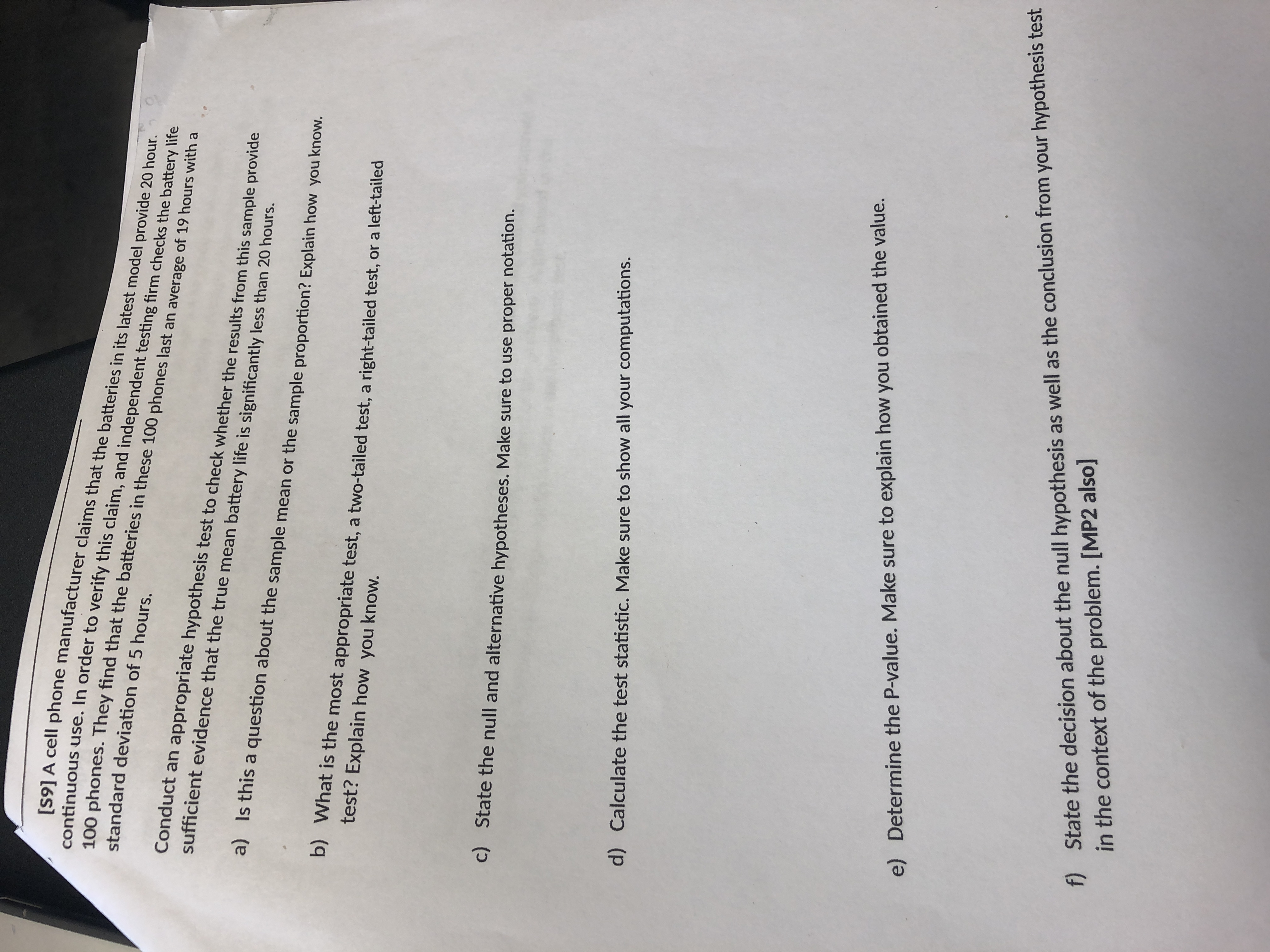# [S9] A cell phone manufacturer claims that the batteries in its latest model provide 20 hour.continuous use. In order to verify this claim, and independent testing firm checks the battery life100 phones. They find that the batteries in these 100 phones last an average of 19 hours with astandard deviation of 5 hours.Conduct an appropriate hypothesis test to check whether the results from this sample providesufficient evidence that the true mean battery life is significantly less than 20 hours.a)Is this a question about the sample mean or the sample proportion? Explain how you know.b)What is the most appropriate test, a two-tailed test, a right-tailed test, or a left-tailedtest? Explain how you know.State the null and alternative hypotheses. Make sure to use proper notation.c)Calculate the test statistic. Make sure to show all your computations.d)Determine the P-value. Make sure to explain how you obtained the value.e)f)State the decision about the null hypothesis as well as the conclusion from your hypothesis testin the context of the problem. [MP2 also]

Question
125 viewshelp_outlineImage Transcriptionclose[S9] A cell phone manufacturer claims that the batteries in its latest model provide 20 hour. continuous use. In order to verify this claim, and independent testing firm checks the battery life 100 phones. They find that the batteries in these 100 phones last an average of 19 hours with a standard deviation of 5 hours. Conduct an appropriate hypothesis test to check whether the results from this sample provide sufficient evidence that the true mean battery life is significantly less than 20 hours. a) Is this a question about the sample mean or the sample proportion? Explain how you know. b) What is the most appropriate test, a two-tailed test, a right-tailed test, or a left-tailed test? Explain how you know. State the null and alternative hypotheses. Make sure to use proper notation. c) Calculate the test statistic. Make sure to show all your computations. d) Determine the P-value. Make sure to explain how you obtained the value. e) f) State the decision about the null hypothesis as well as the conclusion from your hypothesis test in the context of the problem. [MP2 also] fullscreen
check_circle

Step 1

a)

From the given information, the claim of the problem is testing whether the mean battery life is significantly less than 20 hours or not. That is, the testing is for population and which is obtained from sample mean. Hence, the given question is about sample mean.

b)

From the given claim, the hypothesis of the test is ‘less than’ case. Hence, the appropriate test is left-tailed test.

c)

Null Hypothesis:

H0: µ≥20.

That is, there is no sufficient evidence that the true mean battery life is significantly less than 20 hours.

Alternative Hypothesis:

Ha: µ<20.

That is, there is a sufficient evidence that the true mean battery life is significantly less than 20 hours.

Step 2

d)

The value of test statistic is -2 and it is obtained below:

From the given information, the sample mean is 19 and the standard deviation is 5. The sample size is 100.

Step 3

e)

The P-value is 0.0241 and it is calculated below:

The number of sample observations is n=100 and consider the level of significance as 0.05.

The degree of freedom is, – 1 = ...

### Want to see the full answer?

See Solution

#### Want to see this answer and more?

Solutions are written by subject experts who are available 24/7. Questions are typically answered within 1 hour.*

See Solution
*Response times may vary by subject and question.
Tagged in

### Statistics